# MCQs on Control Systems

##### Page 16 of 23. Go to page 1 2 3 4 5 6 7 8 9 10 11 12 13 14 15 16 17 18 19 20 21 22 23
01․ The angle condition used for checking whether any point lies on root locus or not is...........
± (2q + 1) 180o.
± (2q) 180o.
± (2q + 1) 360o.
± (2q) 360o.

The root locus is defined as the locus of closed loop poles obtained when system gain k is varied from 0 to ∞. The angle condition is used for checking whether any point lies on root locus or not and also validity of the root locus shape.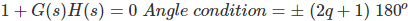The angle condition may be stated as: for a point to lie on root locus the angle evaluated at that point must be an odd multiple of ±180o.

02․ The magnitude condition for root locus is ..................
|G(s)H(s)| = 0
|G(s)H(s)| = 2
|G(s)H(s)| = 1
|G(s)H(s)| = ∞

The magnitude condition is used for finding the value of system gain k at any point on root locus. 1 + G(s)H(s) = 0 G(s)H(s) = -1 + j0 |G(s)H(s)| = √(1² + 0)) |G(s)H(s)| = 1

03․ Consider a point S = -3 + j4 in the s-plane. Then for a system with the open loop transfer function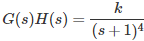?
S is not on root locus
S is on root locus
either 1 or 2
none of the above

The angle condition is used for checking whether any point lies on root locus or not and also validity of the root locus shape.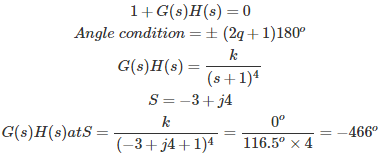∴ - 466° is not the odd multiple of 180°. Therefore, given point is not on the root locus.

04․ A unity feedback control system has an open loop transfer function G(s) = k/(s(s² + 7s + 12) The gain k for which s = -1 + j1 will lie on the root locus of the system is
4
6.5
5
10

The magnitude condition is used for finding the value of system gain k at any point on root locus. 1 + G(s)H(s) = 0 G(s)H(s) = -1 + j0 |G(s)H(s)| = √(1² + 0)) |G(s)H(s)| = 1 |G(s)| at given point = k/(√2*√50) = 1 k = 10

05․ Let P = Number of open loop poles and Z = Number of open loop zeros and P > Z, find the number of branches terminating at ∞?
Z
P - Z
P
Any of the above

The root locus is defined as the locus of closed loop poles obtained when system gain k is varied from 0 to ∞. The root locus is symmetrical about real axis. Let P = Number of open loop poles and Z = Number of open loop zeros and P > Z. The number of branches of root locus = P. The number of branches terminating at zeros = Z. The number of branches terminating at infinity = P – Z.

06․ The characteristic equation of a control system is given by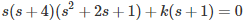What are the angles of asymptotes of the root loci for k ≥ 0?
0°, 120°, 240°
0°, 180°, 300°
60°, 180°, 300°
120°, 180°, 240°

Given characteristic equation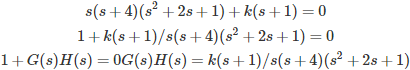Number of poles P = 4 Number of zeros Z = 1, P - Z = 3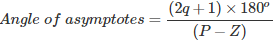∴ Angles of asymptotes for the root loci for k ≥ 0 are 60o, 180oand 300o.

07․ The characteristic equation of a control system is give by s(s + 4)(s² + 2s + 1) + k(s + 1) = 0. Find the angle between the asymptotes?
60°
360°
120°
180°

Given characteristic equation s(s + 4)(s² + 2s + 1) + k(s + 1) =0 1 + k(s + 1) / s(s + 4)(s² + 2s + 1) = 0 Number of poles P = 4 Number of zeros Z = 1 P - Z = 3 Angle between the asymptotes = 2π/(P - Z) = 360°/3 = 120°

08․ Which of the following statements is/are true?
Centroid may be a part of root locus
Centroid may not be a part of root locus
both 1 and 2
nether 1 nor 2

Centroid is the intersection point of the asymptotes on the real axis. It may or may not be a part of the root locus. Centroid =(Σ(Real part of open loop poles) - Σ(Real part of open loop zeros))/(P - Z)

09․ The characteristic equation of a feedback control system is given by s³ + 5s² + (k + 6)s + k = 0. In the root loci diagram, the asymptotes of the root loci for large 'k' meet at a point in the s-plane whose coordinates are
(2,0)
(-2,0)
(-1,0)
(1,0)

Given characteristic equation s³ + 5s² + (k + 6)s + k = 0 1 + k(s + 1)/(s³ + 5s² + 6s) = 0 1 + G(s)H(s) = 0 G(s)H(s) = k(s + 1)/(s³ + 5s² + 6s) = k(s + 1)/((s(s + 2)(s + 3)) Number of poles P = 3 Number of zeros Z = 1 P - Z = 2 Centroid =(Σ(Real part of open loop poles) - Σ(Real part of open loop zeros))/(P - Z) Centroid = (0-2-3-(-1))/2 = -4/2 Centroid = (-2,0)

10․ A control system has open loop transfer function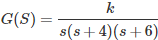. Break away point is lies between
0 and -4.
0 and 4.
-4 and -6.
any of the above.

A point on real axis is said to be on root locus if to the right side of point , the sum of open loop poles and zeros is odd number. When ever there are two adjacently placed poles on the real axis with the section of real axis between them as a part of root locus then there exists break away point between the adjacently placed poles.

<<<1415161718>>>